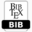## Numerical Approach of a Nonlinear Forward-backward Equation

 AUTHOR(S): M. Filomena Teodoro TITLE Numerical Approach of a Nonlinear Forward-backward EquationPDFBIBTEX ABSTRACT This paper contains a computational approximation for the solution of a forward-backward differential equation that models nerve conduction in a myelinated axon. We look for a solution of an equation defined in R, which tends to known values at ±∞. Extending the approach introduced in [5, 10, 6] for linear case, a numerical method for the solution of problem, is adapted to non linear case using a continuation method 1. KEYWORDS Mixed-type functional differential equations, Non linear Boundary Value Problem, Nerve Conduction, Collocation, Continuation Method, Numerical Approximation, Method of Steps. REFERENCES  K.A. Abell, C.E. Elmer, A.R. Humphries, E.S. VanVleck, Computation of mixed type functional differential boundary value problems, SIADS - Siam J App Dyn Syst 4, 3, 2005, pp. 755-781.  K.A. Abell, C.E. Elmer, A.R. Humphries, E.S. VanVleck, Computation of mixed type functional differential boundary value problems, SIADS - Siam J App Dyn Syst 4, 3, 2005, pp. 755-781.  J. Bell, Behaviour of some models of myelinated axons, IMA J Math App in Med and Biol 1, 2, 1984, pp. 149-167.  H. Chi, J. Bell, B. Hassard, Numerical solution of a nonlinear advance-delay-differential equation from nerve conduction, J Math Biol 24, 1986, pp. 583-601.  V. Iakovleva and C. Vanegas, On the Solution of differential equations withe delayed and advanced arguments, Elect. J Diff Eq Conf 13, 2005, pp. 57-63.  P.M. Lima, M.F. Teodoro, N.J. Ford, P.M. Lumb, Analytical and Numerical Investigation of Mixed Type Functional Differential Equations, J Comp App Math 234, 9, 2010, pp. 2826-2837.  P.M. Lima, M.F. Teodoro, N.J. Ford, P.M. Lumb, Finite Element Solution of a Linear Mixed-Type Functional Differential Equation, Num Alg 55, 2, 2010, pp. 301-320.  P.M. Lima, M.F. Teodoro, N.J. Ford, P.M. Lumb, in: S. Pinelas et al. (Ed.) Analysis and Computational Approximation of a Forward-Backward Equation Arising in Nerve Conduction, ICCEAD 2011, Springer Proc Math Stat NY 2013 47, pp. 475-485.  L.S. Pontryagin, R.V. Gamkreledze, E.F. Mischenko, The Mathematical Theory of Optimal Process, Interscience, New York, 1962.  A. Rustichini, Functional differential equations of mixed type: The linear autonomous case, J Dyn Diff Eq 1, 2, 1989, pp. 121–143.  M.F. Teodoro, P.M. Lima, N.J. Ford, P.M. Lumb, New approach to the numerical solution of forwardbackward equations, Front Math China 4, 1, 2009, pp. 155-168.  M.F. Teodoro, P.M. Lima, N.J. Ford, P.M. Lumb, Numerical modelling of a functional differential equation with deviating arguments using a collocation method, In: T. E. Simos (Ed.), ICNAAM 2008, AIP Proc, Melville-NY, 2008 48, pp. 533-537. Cite this paper M. Filomena Teodoro. (2016) Numerical Approach of a Nonlinear Forward-backward Equation. International Journal of Mathematical and Computational Methods, 1, 75-78Copyright © 2016 Author(s) retain the copyright of this article.This article is published under the terms of the Creative Commons Attribution License 4.0